# 2.7: Transition Metals and Coordination Chemistry (Exercises)

•• OpenStax
• OpenStax
$$\newcommand{\vecs}{\overset { \rightharpoonup} {\mathbf{#1}} }$$ $$\newcommand{\vecd}{\overset{-\!-\!\rightharpoonup}{\vphantom{a}\smash {#1}}}$$$$\newcommand{\id}{\mathrm{id}}$$ $$\newcommand{\Span}{\mathrm{span}}$$ $$\newcommand{\kernel}{\mathrm{null}\,}$$ $$\newcommand{\range}{\mathrm{range}\,}$$ $$\newcommand{\RealPart}{\mathrm{Re}}$$ $$\newcommand{\ImaginaryPart}{\mathrm{Im}}$$ $$\newcommand{\Argument}{\mathrm{Arg}}$$ $$\newcommand{\norm}{\| #1 \|}$$ $$\newcommand{\inner}{\langle #1, #2 \rangle}$$ $$\newcommand{\Span}{\mathrm{span}}$$ $$\newcommand{\id}{\mathrm{id}}$$ $$\newcommand{\Span}{\mathrm{span}}$$ $$\newcommand{\kernel}{\mathrm{null}\,}$$ $$\newcommand{\range}{\mathrm{range}\,}$$ $$\newcommand{\RealPart}{\mathrm{Re}}$$ $$\newcommand{\ImaginaryPart}{\mathrm{Im}}$$ $$\newcommand{\Argument}{\mathrm{Arg}}$$ $$\newcommand{\norm}{\| #1 \|}$$ $$\newcommand{\inner}{\langle #1, #2 \rangle}$$ $$\newcommand{\Span}{\mathrm{span}}$$$$\newcommand{\AA}{\unicode[.8,0]{x212B}}$$

1. Write the electron configurations for each of the following elements:

a. Sc

Let's start off by identifying where Scandium sits on the periodic table: row 4, group 3. This identification is the critical basis we need to write its electron configuration.

By looking at Scandium's atomic number, 21, it gives us both the number of protons and the number of electrons. At the end of writing its electron configuration, the electrons should add up to 21.

At row 4, group 3 Sc, is a transition metal; meaning that its electron configuration will include the D orbital.

Now, we can begin to assign the 21 electrons of Sc to orbitals. As you assign electrons to their orbitals, you move right across the periodic table.

Its first 2 electrons are in the 1s orbital which is denoted as

1s2

where the "1" preceding the s denotes the fact that it is of row one, and it has an exponent of 2 because it fulfills the s orbital's maximum electron number. Now we have 21-2=19 more electrons to assign.

Its next 2 electrons are in the 2s orbital which is denoted as

2s2

where the "2" preceding the s indicates that it is of row two, and it has an exponent of 2 because it fulfills the s orbital's maximum electron number. Now we have 19-2=17 more electrons to assign.

Its next 6 electrons are in the 2p orbital which is denoted as

2p6

where the "2" preceding the p indicates that it is of row two, and it has an exponent of 6 because it fulfills the p orbital's maximum electron number. Now we have 17-6=11 more electrons to assign.

Its next 2 electrons are in the 3s orbital which is denoted as

3s2

where the "3" preceding the s indicates that it is of row three, and it has an exponent of 2 because it fulfills the s orbital's maximum electron number. Now we have 11-2=9 more electrons to assign.

Its next 6 electrons are in the 3p orbital which is denoted as

3p6

where the "3" preceding the p indicates that it is of row three, and it has an exponent of 6 because it fulfills the p orbital's maximum electron number. Now we have 9-6=3 more electrons to assign.

Its next 2 electrons are in the 4s orbital which is denoted as

4s2

where the "4" preceding the s indicates that it is of row four, and it has an exponent of 2 because it fulfills the s orbital's maximum electron number. Now we have 3-2=1 more electron to assign.

Its last electron would be alone in the 3 d orbital which is denoted as

3d1

where the "3" preceding the d indicates that, even though it is technically of row 4, by disregarding the first row of H and He, this is the third row and it has an exponent of 1 because there is only 1 electron to be placed in the d orbital. Now we have assigned all of the electrons to the appropriate orbitals and sub-orbitals, so that the final, entire electron configuration is written as:

1s22s22p63s23p64s23d1

This is the long-hand version of its electron configuration.

So for Sc, its short-hand version of its electron configuration would therefore be:

[Ar] 4s23d1

b. Ti

Start off by identifying where Titanium sits on the periodic table: row 4, group 4, meaning it has 22 electrons total. Titanium is one element to the right of the previous problem's Sc, so we will basically use the same method except, in the end, there will be 2 electrons remaining, so therefore the final orbital will be denoted as:

3d2

If needed, look above to the exact steps for how to do it in detail again; the long-hand electron configuration for Titanium will be:

1s22s22p63s23p64s23d2

So for Ti, its short-hand version of its electron configuration would therefore be:

[Ar] 4s23d2

c. Cr

c. Cr

Start off by identifying where Chromium sits on the periodic table: row 4, group 6, that means it has a total of 24 electrons. But first, Cr, along with Mo, Nb, Ru, Rh, Pd, Cu, Sg, Pt and Au, is a special case. You would think that since it has 24 electrons that its configuration would look like:

1s22s22p63s23p64s23d4

which is how we learned it earlier. However, this electron configuration is very unstable because of the fact that there are 4 electrons in its 3 d orbital. The most stable configurations are half-filled (d5) and full orbitals (d10), so the elements with electrons resulting in ending with the d4 or d9 are so unstable that we write its stable form instead, where an electron from the preceding s orbital will be moved to fill the d orbital, resulting in a stable orbital.

If needed, look above to the exact steps for how to do the beginning of the configuration in detail again. However we have to apple the new rule to attain stability so that the long-hand electron configuration for Chromium will be:

1s22s22p63s23p64s13d5

So for Cr, its short-hand version of its electron configuration would therefore be:

[Ar] 4s13d5

d. Fe

Start off by identifying where Iron sits on the periodic table: row 4, group 8, meaning it has 26 electrons total. This is 5 elements to the right of the previous problem's Sc, so we will basically use the same method except, in the end, there will be 6 electrons remaining, so therefore the final orbital will be denoted as:

3d6

If needed, look above to the exact steps for how to do it in detail again; the long-hand electron configuration for Iron will be:

1s22s22p63s23p64s23d6

So for Fe, its short-hand version of its electron configuration would therefore be:

[Ar] 4s23d6

e. Ru

Start off by identifying where Ruthenium sits on the periodic table: row 5, group 8, that means it has a total of 44 electrons. But first, as stated earlier, Ru, along with Cr, Mo, Nb, Rh, Pd, Cu, Sg, Pt and Au, is a special case. You would think that since it has 44 electrons that its configuration would look like:

1s22s22p63s23p64s23d104p65s2 4d6

which is how we learned it earlier. However, this electron configuration is very unstable because of the fact that, even though there are 4 paired electrons, there are also 4 electrons unpaired. This results in a very unstable configuration, so to restore stability, we have to use a configuration that has the most paired electrons, which would be to take an electron from the s orbital and place it in the d orbital to create:

5s14d7

If needed, look above to the exact steps for how to do the beginning of the configuration in detail again. However we have to apple the new rule to attain stability so that the long-hand electron configuration for Ru will be:

1s22s22p63s23p64s23d104p65s14d7

So for Cr, its short-hand version of its electron configuration would therefore be:

[Kr] 5s14d7

2. Write the electron configurations for each of the following elements and its ions:

a. $$Ti$$

Titanium has an atomic number of 22, meaning it has 22 electrons. The noble gas prior to Titanium is Argon. Looking at row 4 of the periodic table, Titanium still has 4 electrons to be placed in orbitals since Argon has 18 electrons that are already placed. The remaining electrons will fill the $$4s$$ orbital and the remaining two electrons will go into the $$3d$$ orbital. [Ar]4s23d2

b. $$Ti^{+2}$$

This is an ion with a plus 2 charge, meaning 2 electrons have been removed. The electrons will be removed from the $$4s$$ orbital and the 2 remaining electrons will be placed in the $$3d$$ orbital. Like number 1, the prior noble gas is Argon. [Ar]3d2

c. $$Ti^{+3}$$

This is an ion with a plus 3 charge, meaning 3 electrons have been removed. The first 2 electrons will be removed from the $$4s$$ orbital, and the third will be taken from the $$3d$$ orbital, and the 1 remaining electron will be placed in the 3d orbital. Like number 1, the prior noble gas is Argon. [Ar]3d1

d. $$Ti^{+4}$$

This is an ion with a plus 4 charge, meaning 4 electrons have been removed. The first 2 electrons will be removed from the $$4s$$ orbital and the second 2 will be removed from the $$3d$$ orbital. This results in the ion having the same electron configuration as Argon. [Ar]

3. Write the electron configurations for each of the following elements and its 3+ ions:

1. La

b. Sm

c. Lu

La: [Xe]6s 25d 1, La3+: [Xe]; Sm: [Xe]6s 24f 6, Sm3+: [Xe]4f 5; Lu: [Xe]6s 24f 145d 1, Lu3+: [Xe]4f 14

4. Indicate the coordination number for the central metal atom in each of the following coordination compounds:

a. [Pt(H2O)2Br2]

a. The 2 aqua and the 2 bromo ligands form a total of 4 coordinate covalent bonds and as a result the coordination number is 4;
b. The ammine, pyridine, chloro and bromo each form one coordinate covalent bond that gives a total of 4 and hence CN=4;
c. Two ammine and two chloro ligands give a total of 4 coordinate covalent bonds and a CN = 4;
d. One ammine, a pyrimidine, a chloro and a bromo ligand give a total of 4 covalent bonds, resulting in CN = 4.;
e. Four aqua ligands and two chloro ligands form a total of 6 coordinate covalent bonds and a CN =6;
f. Ethylenediamine is a bidentate ligand that forms two coordinate covalent bonds and along with two cyano ligands it forma a total of 6 bonds. hence has a CN=6

b. [Pt(NH3)(py)(Cl)(Br)] (py = pyridine, C5H5N)

b. The ammine, pyridine, chloro and bromo each form one coordinate covalent bond that gives a total of 4 and hence CN=4;

c. [Zn(NH3)2Cl2]

c. Two ammine and two chloro ligands give a total of 4 coordinate covalent bonds and a CN = 4;

d. [Zn(NH3)(py)(Cl)(Br)]

d. One ammine, a pyrimidine, a chloro and a bromo ligand give a total of 4 covalent bonds, resulting in CN = 4.;

e. [Ni(H2O)4Cl2]

e. Four aqua ligands and two chloro ligands form a total of 6 coordinate covalent bonds and a CN =6;

f. [Fe(en)2(CN)2]+ (en = ethylenediamine, C2H8N2)

f. Ethylenediamine is a bidentate ligand that forms two coordinate covalent bonds and along with two cyano ligands it forma a total of 6 bonds. hence has a CN=6

5. Give the coordination numbers and write the formulas for each of the following, including all isomers where appropriate:

1. tetrahydroxozincate(II) ion (tetrahedral)
3. dichloroaurate ion (note that aurum is Latin for "gold")
4. diaminedichloroplatinum(II)
5. potassium diaminetetrachlorochromate(III)
6. hexaaminecobalt(III) hexacyanochromate(III)
7. dibromobis(ethylenediamine) cobalt(III) nitrate
To determine coordination numbers we must count the total number of ligands bonded to the central metal and distinguish monodentate and polydentate ligands. To determine the formulas, we use the nomenclature rules and work backwards.
a. "tetrahydroxo" = 4 hydroxide ligands, since hydroxide is a monodentate ligand, we have a total of 4 bonds to the central metal
Coordination Number: ​​​​​​4
Since the charge on Zinc is 2+ which is given in the nomenclature by the roman numerals, we can calculate the total charge on the complex is 2-
Formula: [Zn(OH)4]2−
b. "hexacyano" = 6 cyanide ligands, since cyanide is a monodentate ligand, we have a total of 6 bonds to the central metal
Coordination Number: 6
We review the basics of nomenclature and see that "hexa" = 6 and "cyano" = CN-. Since the charge on Pd is 4+ which is given in the nomenclature by the roman numerals, we can calculate the total charge on the complex is 2-
Formula: [Pd(CN)6]2−
c. "dicholor" = 2 chloride ligands, since chloride is a monodentate ligand, we have a total of 2 bonds to the central metal
Coordination Number: 2
We review the basics of nomenclature and see that "di" = 2 and "chloro" = Cl-. Since the charge on Au is always 1+, we can calculate the total charge on the complex is 1-
Formula: [AuCl2]
d. "diamine" = 2 ammonia ligands and "dichloro" = 2 chloride ligands, since both ammonia and chloride ligands are monodentant, we have a total of 4 bonds to the central metal
Coordination Number: 4
e. 6, [Co(NH3)6][Cr(CN)6];
f. 6, [Co(en)2Br2]NO3

6. Give the coordination number for each metal ion in the following compounds:

1. [Co(CO3)3]3− (note that CO32 is bidentate in this complex)
2. [Cu(NH3)4]2+
3. [Co(NH3)4Br2]2(SO4)3
4. [Pt(NH3)4][PtCl4]
5. [Cr(en)3](NO3)3
6. [Pd(NH3)2Br2] (square planar)
7. K3[Cu(Cl)5]
8. [Zn(NH3)2Cl2]
You can determine a compound's coordination number based on how many ligands are bound to the central atom.
a.  In this compound, Cobalt is the central atom, and it has 3 CO32- molecules attached to it. However, CO32- is a bidentate ligand, which means it binds to the central atom in two places rather than one. This means that the coordination number of [Co(CO3)3]3- is 6. A coordination number of 6 means that the structure is most likely octahedral.
b. In this compound, Copper is the central atom. 4 ammonia molecules are attached to it. This means the coordination number is 4, and the structure is likely tetrahedral.
c. For this compound, we can ignore the (SO4)3 because it is not bound to the central atom. The central atom is cobalt, and it has 4 ammonia molecules and 2 bromine molecules bound to it. The coordination number is 6.
d. There are two compounds here, indicated by the brackets. The central atom for both is platinum. One of them has 4 ammonia molecules attached, and the other has 4 chlorine atoms attached. Both complexes have a coordination number of 4.
e. We can ignore (NO3)3 for this compound. The central atom is Chromium. There are 3 ethylenediamine molecules attached to the chromium. Ethylenediamine is a bidentate ligand, so the coordination number is 6.
f. Palladium is the central atom. 2 ammonia molecules and 2 bromine atoms are bound to the palladium atom. The coordination number is 4.
g. We can ignore the K3 structure. Copper is the central atom, and there are 5 chlorine molecules attached to it. The coordination number is 5, so the structure is either trigonal bipyramidal or square pyramidal.
h. In this compound, zinc is the central atom. There are 2 ammonia molecules and 2 chlorine atoms attached. This means that the coordination number is 4.

7. Sketch the structures of the following complexes. Indicate any cis, trans, and optical isomers.

1. [Pt(H2O)2Br2] (square planar)
2. [Pt(NH3)(py)(Cl)(Br)] (square planar, py = pyridine, C5H5N)
3. [Zn(NH3)3Cl]+ (tetrahedral)
4. [Pt(NH3)3Cl]+ (square planar)
5. [Ni(H2O)4Cl2]
6. [Co(C2O4)2Cl2]3− (note that $$\ce{C2O4^2-}$$ is the bidentate oxalate ion, $$\ce{−O2CCO2-}$$)
Hint
Cis and trans are a type of geometric isomer, meaning there is a difference in the orientation in which the ligands are attached to the central metal. In cis, two of the same ligands are adjacent to one another and in trans, two of the same ligands are directly across from one another.
Optical isomers → have the ability to rotate light, optical isomers are also chiral. Only chiral complexes have optical isomers
Chiral → asymmetric, structure of its mirror image is not superimposable
Enantiomers: chiral optical isomers (compound can have multiple enantiomers)
Tetrahedral complex with 4 distinct ligands → always chiral
For tetrahedral, if 2 ligands are the same, then it cannot be chiral, has a plane of symmetry
Solutions:
a. $$[Pt(H_2O)_2Br_2]$$ (square planar)
This complex has 2 kinds of ligands. The matching ligands can either be adjacent to each other and be cis, or they can be across from each other and be trans.b. $$[Pt(NH_3)(py)(Cl)(Br)]$$ (square planar, py = pyridine, $$C_5H_5N$$)
This complex has 4 different ligands. There is no plane of symmetry in any of the enantiomers, making the structures chiral and therefore has optical isomers.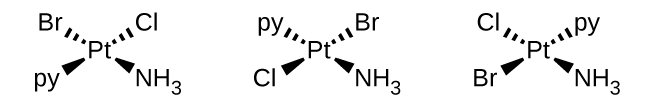c. $$[Zn(NH_3)_3Cl]^+$$ (tetrahedral)
There is a plane of symmetry from $$NH_3$$ through $$Zn$$ to the other $$NH_3$$, therefore it is not chiral.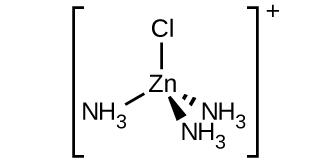d. $$[Pt(NH_3)_3Cl]^+$$ (square planar)
There is a plane of symmetry from $$NH_3$$ through $$Pt$$ to the other $$NH_3$$, therefore it is not chiral.e. $$[Ni(H_2O)_2Cl_2]$$
The $$Cl$$ ligands can either be right next to each other, or directly across from one another allowing for both cis and trans geometries.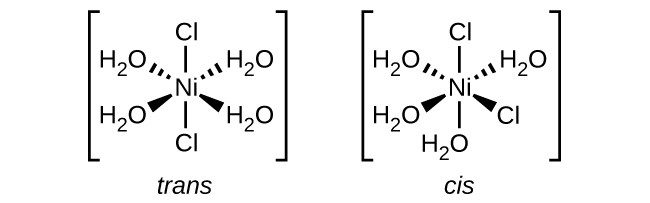f. $$[Co(C_2O_4)_2Cl_2]^-3$$ (note that $$C_2O_4^-2$$ is the bidentate oxalate ion, $$^−O_2CCO_2^-$$
There is a plane of symmetry from $$Cl$$ through Co to the other $$Cl$$ in a "trans" chlorine configuration, therefore it is not chiral in a chlorine "trans" configuration. However, there is no symmetry in the chlorine "cis" configuration, indicating multiple "cis" isomers.8. Draw diagrams for any cis, trans, and optical isomers that could exist for the following (en is ethylenediamine):

1. [Co(en)2(NO2)Cl]+
2. [Co(en)2Cl2]+
3. [Pt(NH3)2Cl4]
4. [Cr(en)3]3+
5. [Pt(NH3)2Cl2]
Hint
We are instructed to draw all geometric isomers and optical isomers for the specified compound. Optical isomers exist when an isomer configuration is not superimposable on its mirror image. This means there are two distinct molecular shapes. Often a left and right hand are cited as an example; if you were to take your right hand and place it upon your left, you cannot make the major parts of your hand align on top of one another. The basic idea when deciding whether something is optically active is to look for a plane of symmetry--if you are able to bisect a compound in a manner that establishes symmetry, then the compound does not have an optical isomer.
Cis isomers exist when there are 2 ligands of the same species placed at 90 degree angles from each other. Trans isomers exist when there are 2 ligands of the same species placed at 180 degree angles from each other.
Problem 1
a. This compound is an octahedral molecule, so the six ligands (atoms in the complex that are not the central transition metal) are placed around the central atom at 90 degree angles. Two optical isomers exist for [Co(en)2(NO2)Cl]+. The second isomer is drawn by taking the mirror image of the first.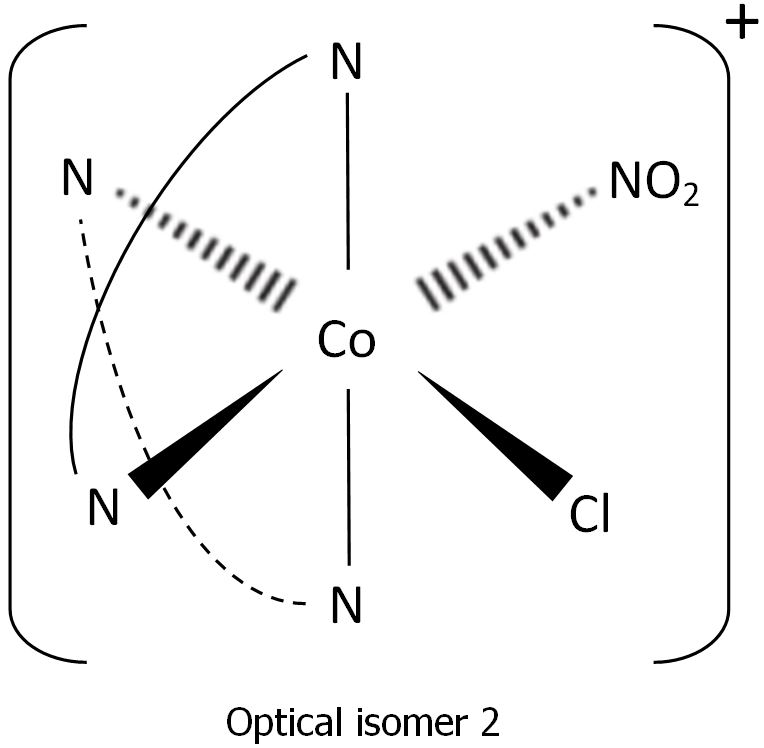b. This compound is also an octahedral molecule. Two cis (optical) isomers and one trans isomer exist for [Co(en)2Cl2]+. The trans isomer can be drawn by placing the chlorine ligands in positions where they form a 180 degree angle with the central atom. The first cis isomer can be drawn by placing the chlorine ligands in positions where they form a 90 degree angle with the central atom. The second cis isomer can be found by mirroring the first cis isomer, like we did in a.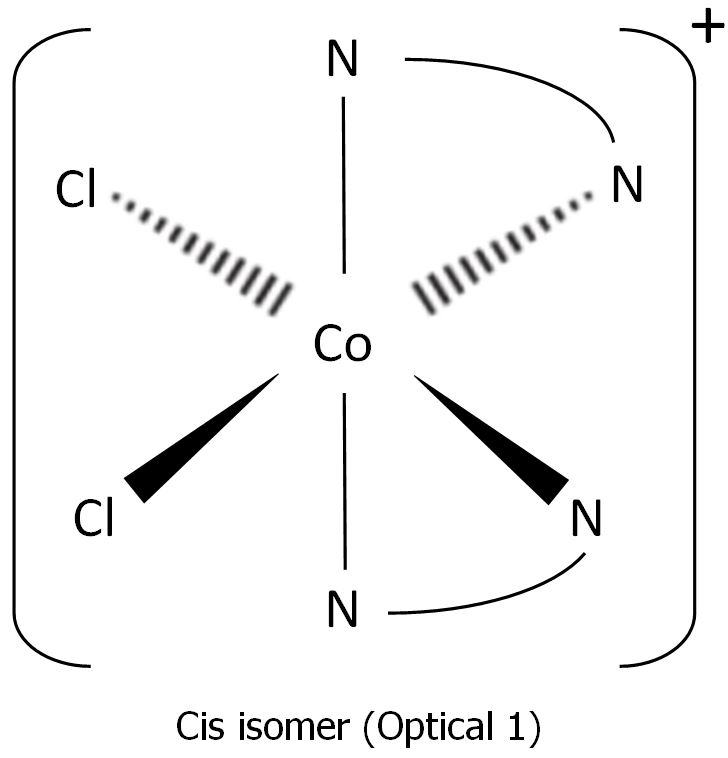c. This compound is also an octahedral molecule. One trans isomer and one cis isomer of [Pt(NH3)2Cl4] exist. The trans isomer can be drawn by placing the ammonia ligands in positions where they form a 180 degree angle with the central atom. The cis isomer can be drawn by placing the ammonia ligands in positions where they form a 90 degree angle with the central atom.d. This compound is also an octahedral molecule. Two optical isomers for [Cr(en)3]3+ exist. The second optical isomer can be drawn by taking the mirror image of the first optical isomer.e. This compound is a square planar complex, so the ligands are placed around the central atom in a plane, at 90 angles. A trans isomer and a cis isomer exist for the complex [Pt(NH3)2Cl2]. The trans isomer can be drawn by placing the ammonia ligands in positions where they form a 180 degree angle in the plane with the central atom. The cis isomer can be drawn by placing the ammonia ligands in positions where they form a 90 degree angle in the plane with the central atom.9. Name each of the compounds or ions given in Exercise 6, including the oxidation state of the metal.

Hint
Rules to follow for coordination complexes
1. Cations are always named before the anions.
2. Ligands are named before the metal atom or ion.
3. Ligand names are modified with an ‐o added to the root name of an anion. For neutral ligands the name of the molecule is used, with the exception of OH2, NH3, CO and NO.
4. The prefixes mono‐, di‐, tri‐, tetra‐, penta‐, and hexa‐ are used to denote the number of simple ligands.
5. The prefixes bis‐, tris‐, tetrakis‐, etc., are used for more complicated ligands or ones that already contain di‐, tri‐, etc.
6. The oxidation state of the central metal ion is designated by a Roman numeral in parentheses.
7. When more than one type of ligand is present, they are named alphabetically. Prefixes do not affect the order.
8. If the complex ion has a negative charge, the suffix –ate is added to the name of the metal.
9. In the case of complex‐ion isomerism the names cis, trans, fac, or mer may precede the formula of the complex‐ion name to indicate the spatial arrangement of the ligands. Cis means the ligands occupy adjacent coordination positions, and trans means opposite positions just as they do for organic compounds. The complexity of octahedral complexes allows for two additional geometric isomers that are peculiar to coordination complexes. Fac means facial, or that the three like ligands occupy the vertices of one face of the octahedron. Mer means meridional, or that the three like ligands occupy the vertices of a triangle one side of which includes the central metal atom or ion.
a. tricarbonatocobaltate(III) ion;
b. tetraaminecopper(II) ion;
c. tetraaminedibromocobalt(III) sulfate;
d. tetraamineplatinum(II) tetrachloroplatinate(II);
e. tris-(ethylenediamine)chromium(III) nitrate;
g. potassium pentachlorocuprate(II);
h. diaminedichlorozinc(II)

10. Name each of the compounds or ions given in Exercise 8.

a. Chlorobis(ethylenediamine)nitrocobalt(III)
b. Dichlorobis(Ethylenediamine)cobalt(III)
c. Diamminetetrachloroplatinum(IV)
d. Tris(ethylenediamine)cromium(III)
e. Diamminedichloroplatinum(II)

11. Specify whether the following complexes have isomers.

1. tetrahedral [Ni(CO)2(Cl)2]
2. trigonal bipyramidal [Mn(CO)4NO]
3. [Pt(en)2Cl2]Cl2
a. none;
b. none;
c. The two Cl ligands can be cis or trans. When they are cis, there will also be an optical isomer.

12. Predict whether the carbonate ligand $$\ce{CO3^2-}$$ will coordinate to a metal center as a monodentate, bidentate, or tridentate ligand.

CO3-2 will coordinate to a metal center as a monodentate ligand.

13. Draw the geometric, linkage, and ionization isomers for [CoCl5CN][CN].

Hint
Isomers are compounds with same formula but different atom arrangement. There are two subcategories: structural isomers, which are isomers that contain the same number of atoms of each kind but differ in which atoms are bonded to one another, and stereoisomers, isomers that have the same molecular formula and ligands, but differ in the arrangement of those ligands in 3D space.
There are three subcategories under structural isomers: ionization isomers, which are isomers that are identical except for a ligand has exchanging places with an anion or neutral molecule that was originally outside the coordination complex; coordination isomers, isomers that have an interchange of some ligands from the cationic part to the anionic part; and linkage isomers, in two or more coordination compounds in which the donor atom of at least one of the ligands is different.
There are also two main kinds of stereoisomers: geometric isomers, metal complexes that differ only in which ligands are adjacent to one another (cis) or directly across from one another (trans) in the coordination sphere of the metal, and optical isomers, which occurs when the mirror image of an object is non-superimposable on the original object.
Some of the isomers look almost identical, but that is because the CN ligand can be attached by both (but not at the same time) the C or N.## Spectroscopic and Magnetic Properties of Coordination Compounds

14. Determine the number of unpaired electrons expected for [Fe(NO2)6]3−and for [FeF6]3− in terms of crystal field theory.

Hint
The crystal field theory is is a model that describes the breaking of degeneracies of electron orbital states, usually d or f orbitals, due to a static electric field produced by a surrounding charge distribution.
The degenerate d-orbitals split into two levels, e$$_{g}$$ and t$$_{2g}$$, in the presence of ligands.
The energy difference between the two levels is called the crystal-field splitting energy, $$\Delta_{\circ}$$.
After 1 electron each has been filled in the three t$$_{2g}$$ orbitals, the filling of the fourth electron takes place either in the e$$_{g}$$ orbital or in the t$$_{2g}$$, where the electrons pair up. depending on whether the complex is high spin or low spin.
If the $$\Delta_{\circ}$$ value of a ligand is less than the pairing energy (P), then the electrons enter the e$$_{g}$$ orbital, but if the $$\Delta_{\circ}$$ value of a ligand is more than the pairing energy (P), then the electrons enter the t$$_{2g}$$ orbital.
when the is less than the pairing energy, the electrons prefer then eg orbitals because there is not enough energy to pair the electrons together. It will be high spin
when the is more then the pairing energy, the electrons prefer the t2g because there is enough energy to pair the electrons. It will be low spin.

Step 1: Determine the oxidation state of the Fe
For $$[Fe(NO_{2}){_{6}}]^{3-}$$ and $$[FeF_{6}]^{3-}$$, both $$NO_{2}$$ and $$F_{6}$$ have a charge of -1. Since there is 6 of them then that means the charge is -6 and in order for there to be an overall charge of -3, Fe has to have a +3 charge.
Step 2: Determine type of ligand
Based on the spectrochemical series we can see that $$NO_{2}^{-}$$ is a stronger field ligand than F$$^{-}$$, and therefore is a low spin complex because it has a high $$\Delta_{\circ}$$ unlike F$$^{-}$$ which is a high spin.
Step 3: Draw the crystal field
$$[Fe(NO_{2}){_{6}}]^{3-}$$$$[FeF_{6}]^{3-}$$There is 1 unpaired electron for $$[Fe(NO_{2}){_{6}}]^{3-}$$, and 5 for $$[FeF_{6}]^{3-}$$ based on the crystal field theory.
[Fe(NO2)6]3−:1 electron
[FeF6]3−:5 electrons

15. Draw the crystal field diagrams for [Fe(NO2)6]4− and [FeF6]2−. State whether each complex is high spin or low spin, paramagnetic or diamagnetic, and compare Δoct to P for each complex.16. Give the oxidation state of the metal, number of d electrons, and the number of unpaired electrons predicted for [Co(NH3)6]Cl3.

a. The ox state is 3+, there are 6 d electrons, there are no unpaired electrons

17. The solid anhydrous solid CoCl2 is blue in color. Because it readily absorbs water from the air, it is used as a humidity indicator to monitor if equipment (such as a cell phone) has been exposed to excessive levels of moisture. Predict what product is formed by this reaction, and how many unpaired electrons this complex will have.

[Co(H2O)6]Cl2 with three unpaired electrons.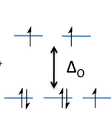18. Is it possible for a complex of a metal in the transition series to have six unpaired electrons? Explain.

It is not possible for a metal in the transition series to have six unpaired electrons. This is because transition metals have a general electron configuration of (n-1)d1-10 ns1-2 where n is the quantum number. The last electron will go into the d orbital which has 5 orbitals that can each contain 2 electrons, yielding 10 electrons total. According to Hund's Rule, electrons prefer to fill each orbital singly before they pair up. This is more energetically favorable. Since there are only 5 orbitals and due to Hund's Rule, the maximum number of unpaired electrons a transition metal can have is 5. Therefore, there cannot be a complex of a transition metal that has 6 unpaired electrons.
For example, lets look at iron's electron configuration. Iron has an electron configuration of 1s22s22p63s23p64s23d6. Now the most important orbital to look at is the d orbital which has 6 electrons in it, but there are only 4 unpaired electrons as you can see by this diagram:
3d: [↿⇂] [↿] [↿][↿][↿]
Each [ ] represents an orbital within the d orbital. This diagram follows Hund's rule and shows why no transition metal can have 6 unpaired electrons.

19. How many unpaired electrons are present in each of the following?

1. [CoF6]3− (high spin)
2. [Mn(CN)6]3− (low spin)
3. [Mn(CN)6]4− (low spin)
4. [MnCl6]4− (high spin)
5. [RhCl6]3− (low spin)
a. 4; b. 2; c. 1; d. 5; e. 0

20. Explain how the diphosphate ion, [O3P−O−PO3]4−, can function as a water softener that prevents the precipitation of Fe2+ as an insoluble iron salt.

The diphosphate ion, [O3P−O−PO3]4− can function as a water softener keeping the iron in a water soluble form because of its more negative electrochemical potential than water's. This is similar to the way plating prevents metals from reacting with oxygen to corrode.
Mineral deposits are formed by ionic reactions. The Fe2+ will form an insoluble iron salt of iron(III) oxide-hydroxide when a salt of ferric iron hydrolyzes water. However, with the addition of [O3P−O−PO3]4−, the Fe2+ cations are more attracted to the PO3 group, forming a Fe(PO3) complex.
The excess minerals in this type of water is considered hard thus its name hard water.

21. For complexes of the same metal ion with no change in oxidation number, the stability increases as the number of electrons in the t2g orbitals increases. Which complex in each of the following pairs of complexes is more stable?

1. [Fe(H2O)6]2+ or [Fe(CN)6]4−
2. [Co(NH3)6]3+ or [CoF6]3−
3. [Mn(CN)6]4− or [MnCl6]4−
Hint
The Spectrochemical Series is as follows $I^{-}<Br^{-}<SCN^{-}\approx Cl^{-}<F^{-}<OH^{-}<ONO^{-}<ox<H_{2}O<SCN^{-}<EDTA<NH_{3}<en<NO_{2}^{-}<CN$
The strong field ligands (on the right) are low spin which fills in more electrons in the t2g orbitals. The weak field ligands (on the left) are high spin so it can fill electrons in the t2g orbitals and eg orbitals. In conclusion, more electrons are filled up from the strong field ligands because the electrons don't move up to the eg orbitals.a. [Fe(CN)6]4−; b. [Co(NH3)6]3+; c. [Mn(CN)6]4−

### Q19.3.9

Trimethylphosphine, P(CH3)3, can act as a ligand by donating the lone pair of electrons on the phosphorus atom. If trimethylphosphine is added to a solution of nickel(II) chloride in acetone, a blue compound that has a molecular mass of approximately 270 g and contains 21.5% Ni, 26.0% Cl, and 52.5% P(CH3)3 can be isolated. This blue compound does not have any isomeric forms. What are the geometry and molecular formula of the blue compound?

### S19.3.9

1)Find the empirical formula. There is a total of 270 grams. To find out how many grams of each element/compound there are, multiply the percentage by the mass (270).

$(270g)(0.215)=58.05gNi$

$(270g)(0.26)=70.2gCl$

$(270g)(0.525)=141.75gP(CH_3)_3$

Now that we have the grams of each element/compound, we can convert them to moles by using their molar mass.

$(58.055gNi)(\frac{1mol.}{58.69gNi})=0.989mol.Ni$

$(70.2gCl)(\frac{1mol.}{35.45gCl})=1.98mol.Cl$

$(141.75gP(CH_3)_3)(\frac{1mol.}{76.07gP(CH_3)_3})=1.86mol.P(CH_3)_3$

Now that we have the moles of all elements/compounds, we can find the ratio of all them to each other. To do this, we take the element/compound with the least amount of moles and divide all element/compound moles by this amount. In this case, Ni has the least number of moles.

$\frac{0.989mol.Ni}{0.989mol.Ni}=1$

$\frac{1.98mol.Cl}{0.989mol.Ni}=approx.2$

$\frac{1.86mol.P(CH_3)_3}{0.989mol.Ni}=approx.2$

We now know the ratio of all element/compounds in the blue compound.

The empirical formula is: NiCl(P(CH3)3)2

This formula shows us there are 4 ligands. There are 2 chlorine ligands and 2 trimethylphosphine ligands. This means that the blue compound has either a tetrahedral or square planar shape, where tetrahedral shapes are capable of different isomeric forms when all ligands are different (because if not, there is only 1 way for them to be arranged), and square planar shapes are capable of cis/trans forms. In the problem, it states this compound does not have any isomeric forms, therefore this has a tetrahedral shape.

### A19.3.9

a) NiCl(P(CH3)3)2

b) Tetrahedral

### Q19.3.10

Would you expect the complex [Co(en)3]Cl3 to have any unpaired electrons? Any isomers?

### S19.3.10

Assign oxidation states to each element. Cl- has a -1 oxidation state. En is neutral, so 0. The entire complex is also neutral, so in order to balance the charges out, Co must be +3 because there are 3 chlorides, which gives a -3 charge.

STEP 2:

Write the electron configuration for $$Co^{3+}$$. $$[Ar]3d^6$$. There are 6 electrons.

STEP 3: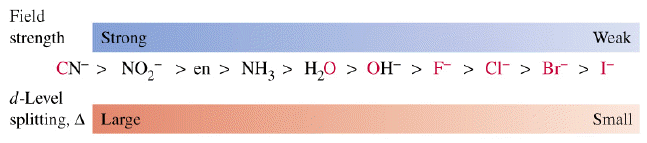Check where en lies on the spectrochemical series. Does it have a strong field strength? It does, so these electrons will exist at the d-level with high splitting energy because the magnitude of the pairing energy is less than the crystal field splitting energy in the octahedral field.

You will the notice that there aren't any unpaired electrons when you draw the Crystal Field Theory (CFT) diagram.This complex does not have any geometric isomers because cis-trans structures cannot be formed. The mirror image is nonsuperimpoasable, which means the enantiomers are chiral molecules; if the mirror image is placed on top on the original molecule, then they will never be perfectly aligned to give the same molecule.

### A19.3.10

The complex does not have any unpaired electrons. The complex does not have any geometric isomers, but the mirror image is nonsuperimposable, so it has an optical isomer.

### Q19.3.11

Would you expect the Mg3[Cr(CN)6]2 to be diamagnetic or paramagnetic? Explain your reasoning.

### S19.3.11

The first step to determine the magnetism of the complex is to calculate the oxidation state of the transition metal. In this case, the transition metal is Cr.

Before doing so, we need to find charge of the of the complex ion [Cr(CN)6]2 given that the oxidation state of Mg3 is 2+. Using the subscripts of the $$\displaystyle Mg^{2+}$$ ion and the [Cr(CN)6]2 complex, we find that the oxidation state of [Cr(CN)6]2 , x, to be:

$$\displaystyle 3(+2)+2(x)=0$$

$$\displaystyle x=3$$

Now that we found the charge of the coordination complex, we are able to find the charge of the transition metal Cr given that the charge of CN is -1. Again, using the subscripts we find the oxidation state of Cr, y, to be:

$$\displaystyle y + 6(-1)=-3$$

$$\displaystyle y=3$$

Therefore, the oxidation state of the transition metal Cr is $$\displaystyle Cr^{3+}$$

Next, using the transition metal $$\displaystyle Cr^{3+}$$ and the periodic table as reference, we can determine the electron configuration of $$\displaystyle Cr^{3+}$$ to be $$\displaystyle [Ar]d^{3}$$. This means that $$\displaystyle Cr^{3+}$$ has 3 unpaired electrons in the 3d sublevel. Therefore, we find that since at least one electron is unpaired(in this case all 3 electrons are unpaired), Mg3[Cr(CN)6]2 is paramagnetic.

A19.3.11

a) Paramagnetic

### Q19.3.12

Would you expect salts of the gold ion, Au+, to be colored? Explain.

### S19.3.12

No. Colored ions have unpaired electrons in their outmost orbital. A partially filled d orbital, for example, can yield various colors. After completing the noble gas configuration, we see that Au+ has a configuration of [Xe] 4f145d10. Since Au+ has a completely filled d sublevel, we are certain that any salts of the gold ion, Au+ will be colorless.

*An example of a colored ion would be copper(II). Cu2+ has an electron configuration of [Ar]3d9. It has one unpaired electron. Copper(II) appears blue.

### A19.3.12

No. Au+ has a complete 5d sublevel.

### Q19.3.13

[CuCl4]2− is green. [Cu(H2O)6]2+is blue. Which absorbs higher-energy photons? Which is predicted to have a larger crystal field splitting?

### S19.3.13

Although a color might appear a certain way, it actual absorbs a different color, opposite of it on the color wheel.In this case;

[CuCl4]2- appears green but is opposite of red on the color wheel which is absorbed and is characterized by wavelengths 620-800 nanometers.

[Cu(H2O)6]2+ appears blue but is opposite of orange on the color wheel which is absorbed and is characterized by wavelengths 580-620 nanometers.

When determining which absorbs the higher energy photons, one must look at the complex itself. A higher energy indicates a high energy photon absorbed and a lower energy indicates a lower energy photon absorbed. How can we determine this? By looking at the complex and more specifically the ligand attached and its location in the spectrochemical series.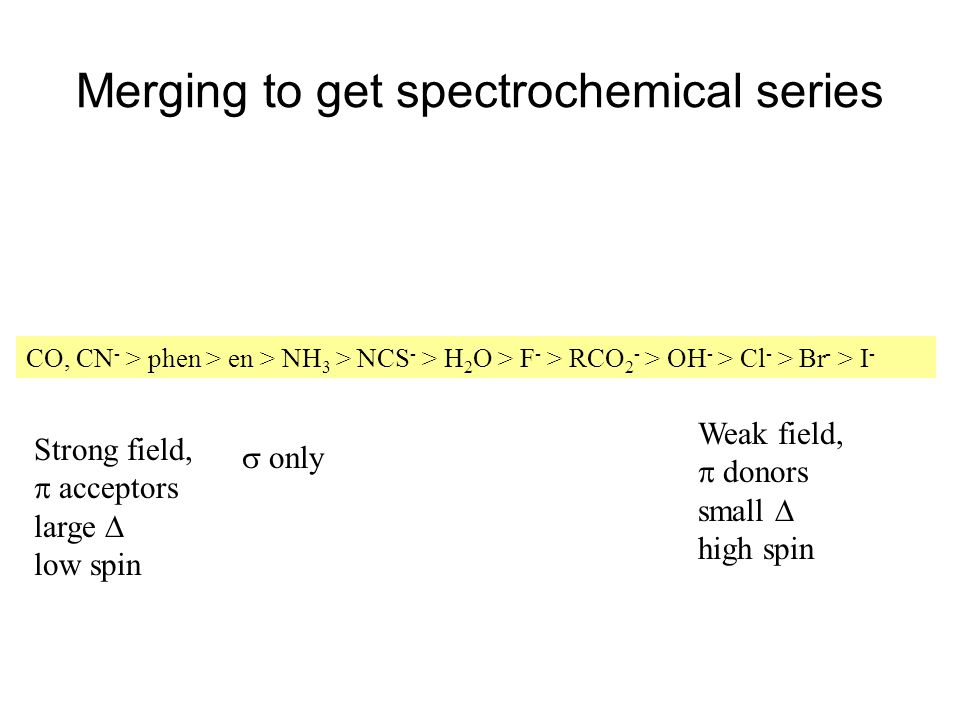The ligands attached are Water and Chlorine and since Water is a stronger ligand than Chlorine according to the series, it also has larger energy, indicating a higher energy. This means that the complex [Cu(H2O)6]2+ absorbs a higher energy photon because of its a stronger ligand than chlorine.

Part 2 of this question also asks which complex is predicted to have a larger crystal field splitting. To determine this you also use the spectrochemical series and see which ligand is stronger. Since H2O is stronger than Cl- on the spectrochemical series, we can say [Cu(H2O)6]2+ has a higher crystal field splitting.

### A19.3.13

a) [Cu(H2O)6]2+

b) [Cu(H2O)6]2+ has a higher crystal field splitting

This page titled 2.7: Transition Metals and Coordination Chemistry (Exercises) is shared under a CC BY license and was authored, remixed, and/or curated by OpenStax.